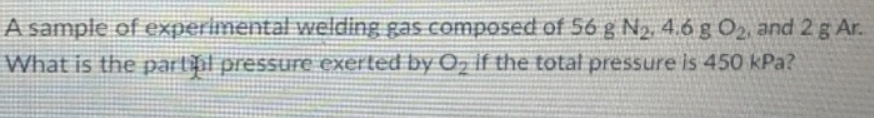# Problem: A sample of experimental welding gas composed of 56 g N2, 4.6 g O2, and 2 g Ar. What is the partial pressure exerted by O2 if the total pressure is 450 kPa?

###### FREE Expert Solution
97% (460 ratings)###### Problem Details

A sample of experimental welding gas composed of 56 g N2, 4.6 g O2, and 2 g Ar. What is the partial pressure exerted by O2 if the total pressure is 450 kPa?